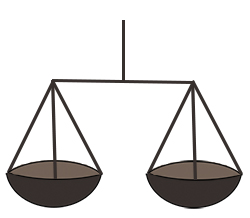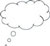In arithmetic, the equals sign is often seen as a command to take an action and find an answer. Therefore, when a student sees an equals sign in an equation, they may want to carry out the operation that precedes it. To many students, the equals sign means ‘and the answer is’, which is not helpful when doing algebra.

The equals sign is always a representation of a relationship between two expressions. The equals sign represents quantitative sameness – in other words, the expression on the left-hand side of the equals sign represents the same quantity as the expression on the right-hand side. The equals sign can be read as ‘is the same as’, or ‘is equivalent to’, or ‘is of equal value’. Understanding this will help your students when they work with equations.Pause for thought Think about some uses of the equals sign that your students may see around them that may lead to a misinterpretation or misunderstanding. For example, the equals sign can sometimes be used outside mathematical equations, such as ‘MATHS = FUN’ or ‘Ravi = 9’.